Open in App
Not now

# Accounting Treatment for Interest on Partner’s Capital

• Last Updated : 29 Jun, 2022

Interest on Capital is an interest allowed to the partners on the capital amount invested by them into the firm. Such interest is not paid in cash, instead the partner’s capital account is credited by the same amount. Interest on Capital is paid by the firm and is therefore an expense for the firm that reduces the profit. However, Interest on Capital is not always charged against the profit directly, rather a new account ‘Profit and Loss Appropriation Account’ is opened.

Interest on Capital is allowed at a fixed rate, agreed by all the partners and mentioned in the Partnership Deed. This also means that in the absence of the deed, no partner is liable to receive such interest on capital. Further, under the concept of appropriation, Interest on Capital is paid only when the firm makes a profit. In case of insufficient profit, i.e., the amount of interest on capital is more than the amount of net profit earned, then interest on capital is allowed maximum up to the amount of the net profit in the ratio of the interest on capital of each partner.

### 1.  When treated as an Appropriation of Profit: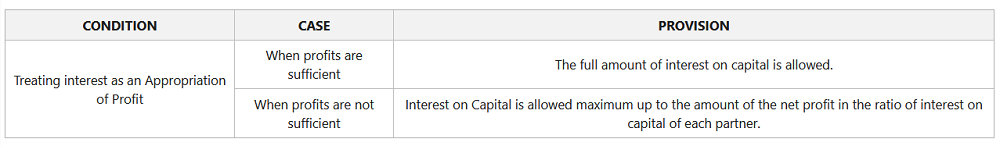Illustration:

Ram and Mohan are partners sharing profit and loss in the ratio of 3:2 with the capital of ₹4,00,000 and ₹2,00,000, respectively. Calculate Interest on capital and show the distribution of the profit under the following cases-

Case A: Calculation of Interest on capital when profits are sufficient:

If the partnership deed provides for the Interest on Capital @ 10% p.a. and the profit for the year is ₹2,00,000.

Solution: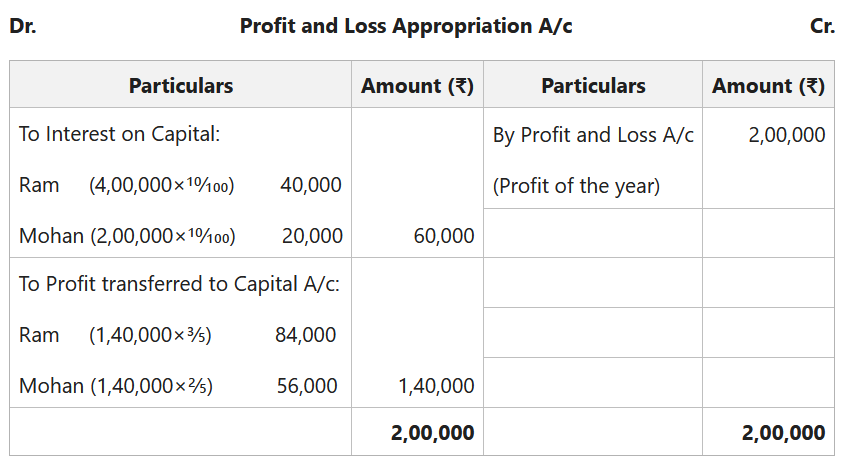Case B: Calculation of Interest on capital when profits are insufficient:

If the partnership deed provides for the Interest on Capital @ 10% p.a. and the profit for the year is ₹30,000.

Solution: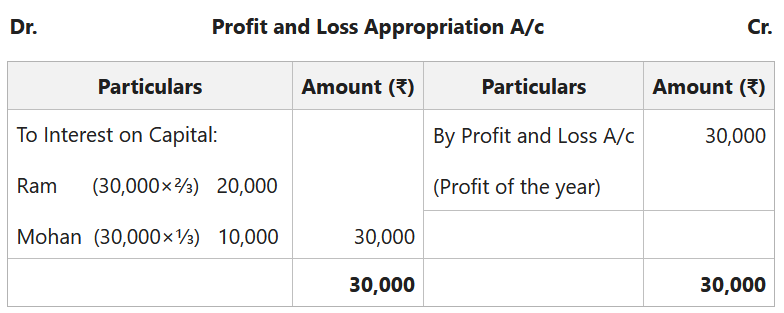Note: The profit available is ₹30,000, whereas the Interest due on capital is ₹60,000 (i.e., ₹40,000+₹20,000). Since the profit is less than the interest, the profit available will be distributed in the ratio of actual interest calculated of each partner, i.e., 40,000:20,000 or 2:1.

### 2. Charge against Profit:

When the Partnership Deed provides that the Interest on Capital shall be charged against the profit, then the full amount of Interest will be allowed whether there is profit or loss.

Illustration:

Ram and Mohan are partners sharing profit and loss in the ratio of 3:2 with a capital of ₹4,00,000 and ₹2,00,000, respectively. Calculate Interest on capital when interest is charged against profit when the profit for the year is 30,000.

Solution: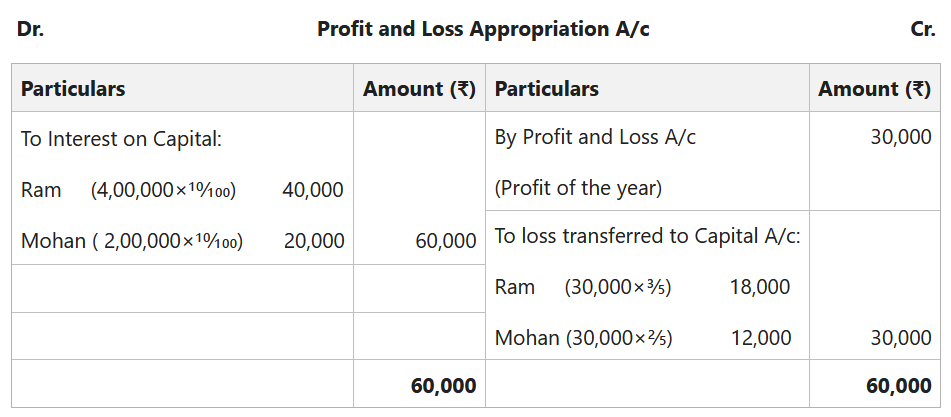#### 3. Interest on Partner’s Capital when Opening Capital is not given:

Interest on Capital is always calculated on the opening capital, hence when opening capital is not given, we need to calculate the opening capital using the following formula:

Opening Capital = Closing Capital + Drawings – Additional Capital – Profit (or + Loss)

Illustration:

Ram and Mohan are partners sharing profit and loss in the ratio of 3:2 with a Closing Capital of ₹5,55,000 and ₹2,80,000, respectively after making all the adjustment, but before charging interest on capital on 31st March 2022. On 1st July 2021, Ram and Mohan withdrew ₹5,000 and ₹10,000, respectively. Further, on 1st January 2022, they invested an additional capital of ₹1,00,000 and ₹50,000, respectively. The profit for the year is 1,00,000. Calculate Interest on Capital when a deed provides for 10%  Interest on Capital charge against profit.

Solution:

Opening Capital = Closing Capital + Drawings – Additional Capital – Profit

For Ram:

Opening Capital = 5,55,000 +5,000 -1,00,000 – 60,000

Opening Capital = ₹4,00,000.

For Mohan:

Opening Capital = 2,80,000 +10,000 -50,000 – 40,000

Opening Capital = ₹2,00,000.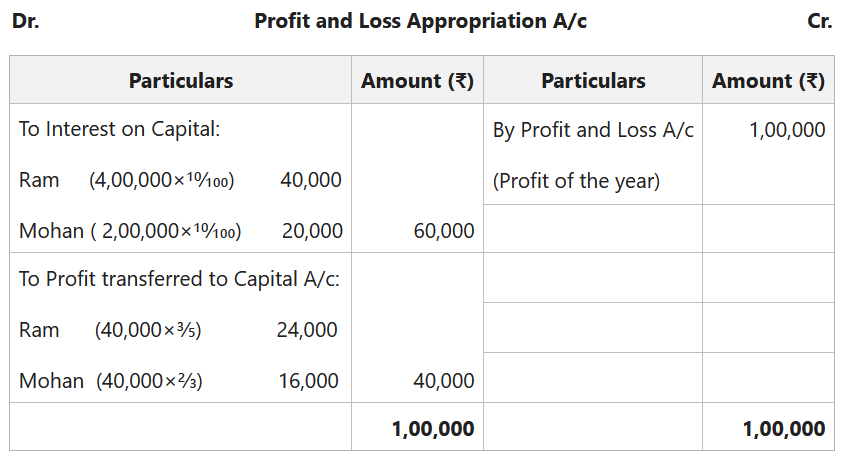#### 4. Interest on Capital under Product Method:

When partners introduce additional capital several times during a year, it is suggested to use the product method to calculate the Interest on Capital.

Product Method: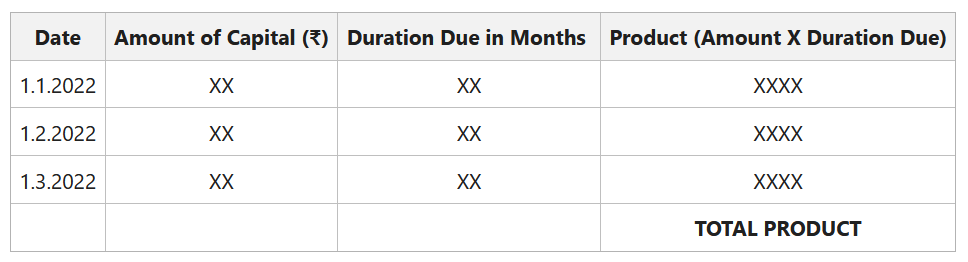Interest on Capital= Total Product×Rate/100×1/12

Illustration:

Ram and Mohan are partners sharing profit and loss in the ratio of 3:2 with a capital of ₹4,00,000 and ₹2,00,000, respectively. Ram made an additional investment of ₹1,00,000 on 1st October 2021 and Mohan on 1st January 2022  further invested ₹50,000. On the same date Ram further introduced ₹50,000 as a capital. If the deed provides for 10% Interest on Capital, calculate the Interest on Capital of both the partners using a Product Method.

Solution:

1. Calculating Interest on Capital of Ram: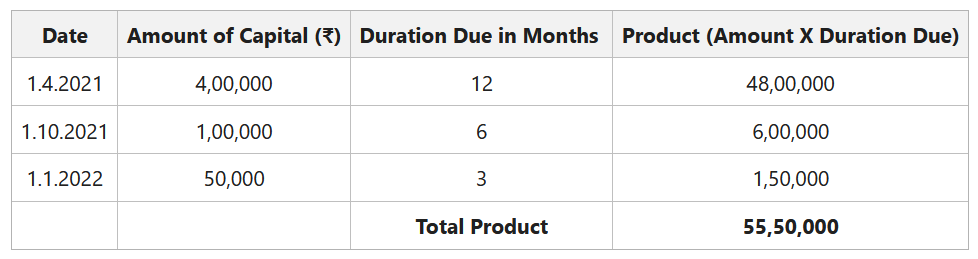Interest on Capital= 55,50,000×10/100×1/12

Interest on Capital =₹46,250

2. Calculating Interest on Capital of Mohan: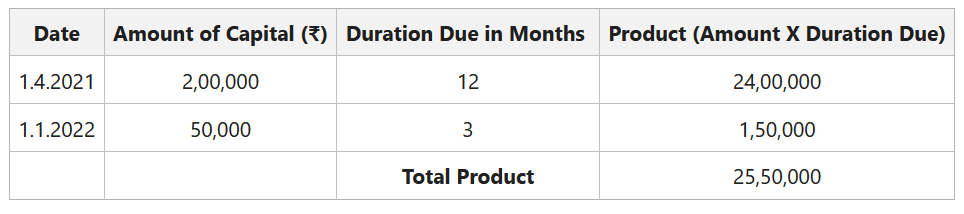Interest on Capital= 25,50,000×10/100×1/12

Interest on Capital= ₹21,250

My Personal Notes arrow_drop_up
Related Articles